×#### Thank you for registering.

One of our academic counsellors will contact you within 1 working day.

Click to Chat

1800-1023-196

+91-120-4616500

CART 0

• 0

MY CART (5)

Use Coupon: CART20 and get 20% off on all online Study Material

ITEM
DETAILS
MRP
DISCOUNT
FINAL PRICE
Total Price: Rs.

There are no items in this cart.
Continue Shopping• Complete JEE Main/Advanced Course and Test Series
• OFFERED PRICE: Rs. 15,900
• View Details

```Revision Notes on Pair of Linear Equations in two variables

Linear Equations

A Linear Equation is an equation of straight line. It is in the form of

ax + by + c = 0

where a, b and c are the real numbers (a≠0 and b≠0) and x and y are the two variables,

Here a and b are the coefficients and c is the constant of the equation.

Pair of Linear Equations

Two Linear Equations having two same variables are known as the pair of Linear Equations in two variables.

a1x + b1y + c1 = 0

a2x + b2y + c2 = 0

Graphical method of solution of a pair of Linear Equations

As we are showing two equations, there will be two lines on the graph.

1. If the two lines intersect each other at one particular point then that point will be the only solution of that pair of Linear Equations. It is said to be a consistent pair of equations.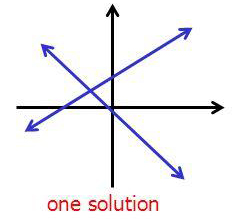2. If the two lines coincide with each other, then there will be infinite solutions as all the points on the line will be the solution for the pair of Linear Equations. It is said to be dependent or consistent pair of equations.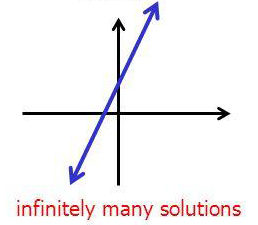3. If the two lines are parallel then there will be no solution as the lines are not intersecting at any point. It is said to be an inconsistent pair of equations.Algebraic Methods of Solving a Pair of Linear Equations

1. Substitution method

If we have a pair of Linear Equations with two variables x and y, then we have to follow these steps to solve them with the substitution method-

Step 1: We have to choose any one equation and find the value of one variable in terms of other variable i.e. y in terms of x.

Step 2: Then substitute the calculated value of y in terms of x in the other equation.

Step 3: Now solve this Linear Equation in terms of x as it is in one variable only i.e. x.

Step 4: Substitute the calculate value of x in the given equations and find the value of y.

2. Elimination method

In this method, we solve the equations by eliminating any one of the variables.

Step 1: Multiply both the equations by such a number so that the coefficient of any one variable becomes equal.

Step 2: Now add or subtract the equations so that the one variable will get eliminated as the coefficients of one variable are same.

Step 3: Solve the equation in that leftover variable to find its value.

Step 4: Substitute the calculated value of variable in the given equations to find the value of the other variable.

3. Cross multiplication method

Given two equations in the form of

a1x + b1y + c1 = 0 and a2x + b2y + c2 = 0, whereWe write it in general form as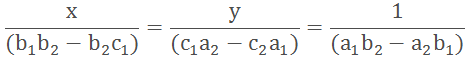To apply cross multiplication we use this diagram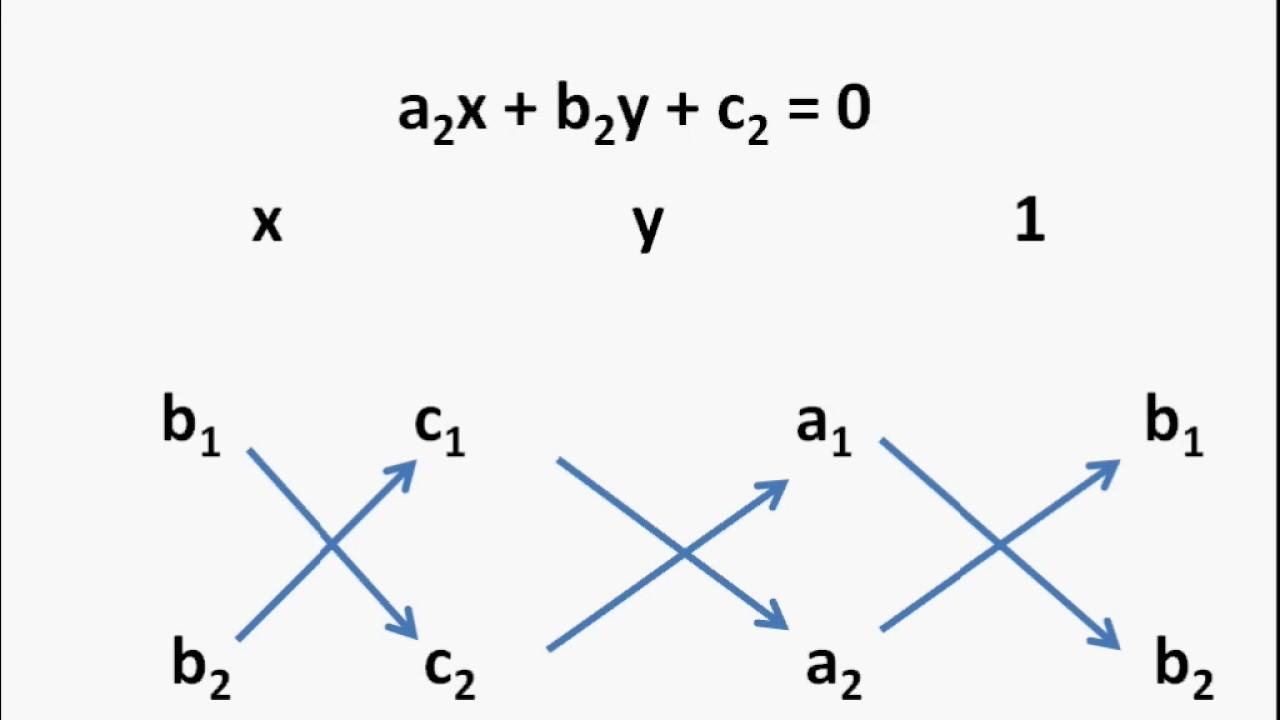The arrows indicate the pairs to be multiplied. The product of the upward arrow pairs is to be subtracted from the product of the downward arrow pairs.

By using this diagram we have to write the equations as given in general form then find the value of x and y by putting the values in the above notations.

y Interpretation of the pair of equations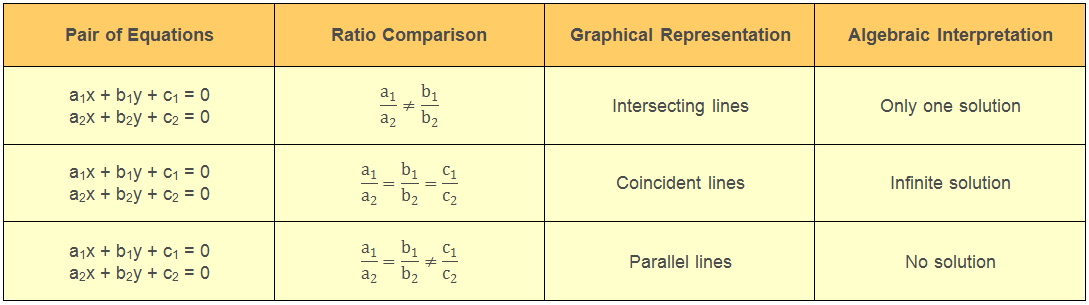Equations Reducible to a Pair of Linear Equations in Two Variables

There are some pair of equations which are not linear but can be reduced to the linear form by substitutions.

Given equations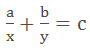We can convert these type of equations in the form of ax + by + c = 0

Let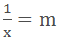and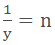Now after substitution the equation will be

am + bn = c

It can be easily solved by any of the method of solving Linear Equations.
```### Course Features

• 728 Video Lectures
• Revision Notes
• Previous Year Papers
• Mind Map
• Study Planner
• NCERT Solutions
• Discussion Forum
• Test paper with Video Solution